# Selina Solutions Concise Maths Class 7 Chapter 9: Profit, Loss and Discount Exercise 9B

Selina Solutions Concise Maths Class 7 Chapter 9 Profit, Loss and Discount Exercise 9B help students understand the important formulas, which are used in finding the cost price of a given object. Various solved examples are present before the exercise problems to help students understand the method of solving them. The solutions have shortcut methods and important formulas are explained in simple language for the students. To improve conceptual knowledge among students, Selina Solutions Concise Maths Class 7 Chapter 9 Profit, Loss and Discount Exercise 9B, PDF links are given here for free download.

## Selina Solutions Concise Maths Class 7 Chapter 9: Profit, Loss and Discount Exercise 9B Download PDF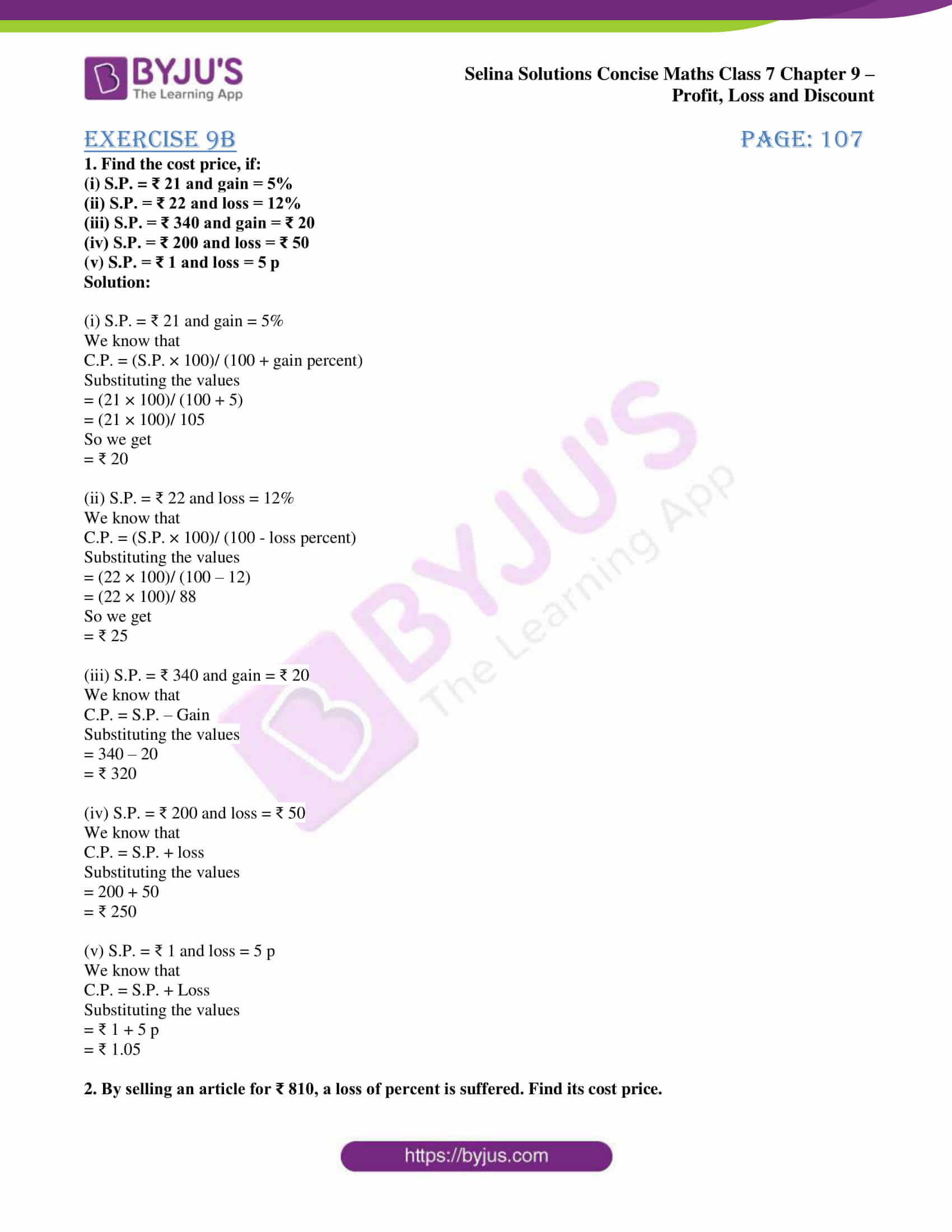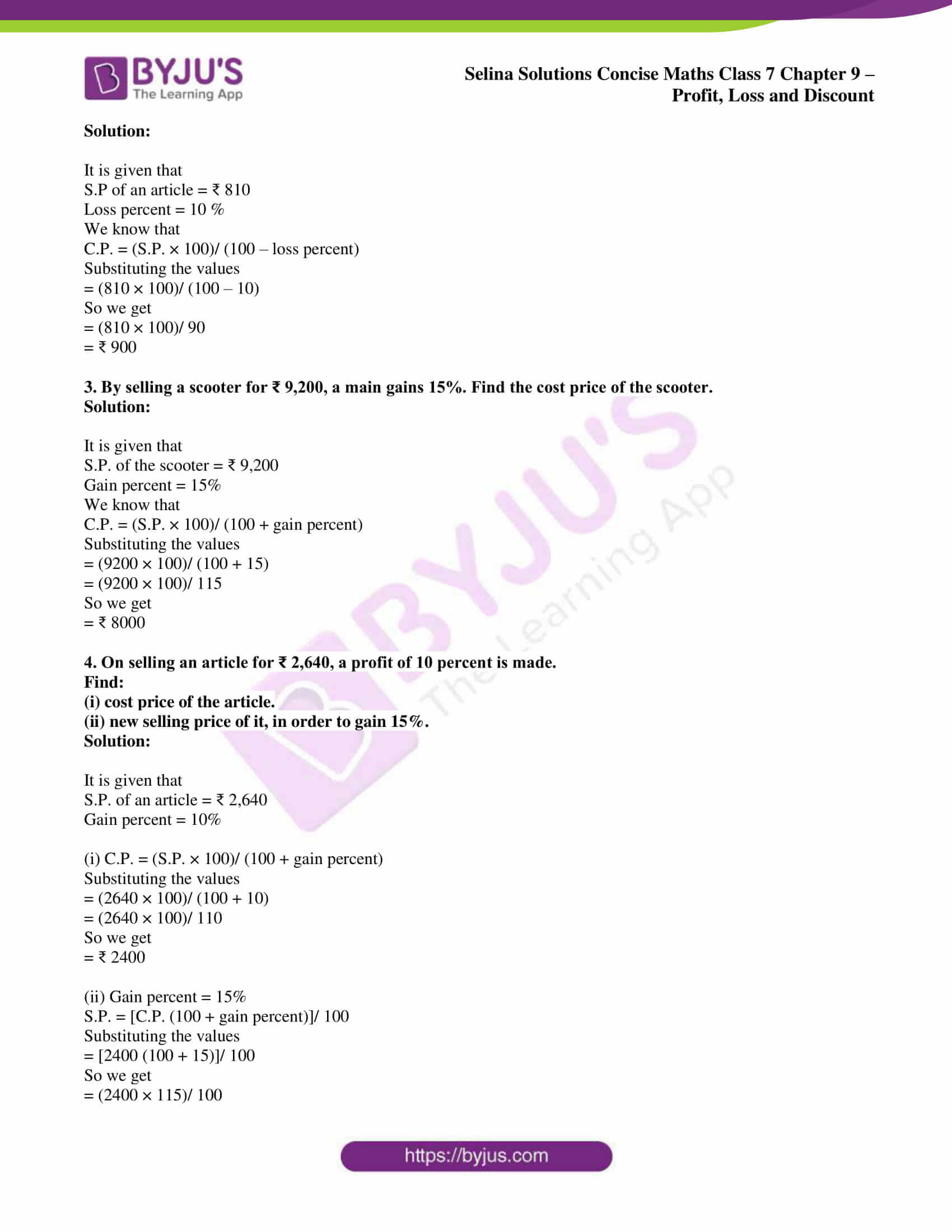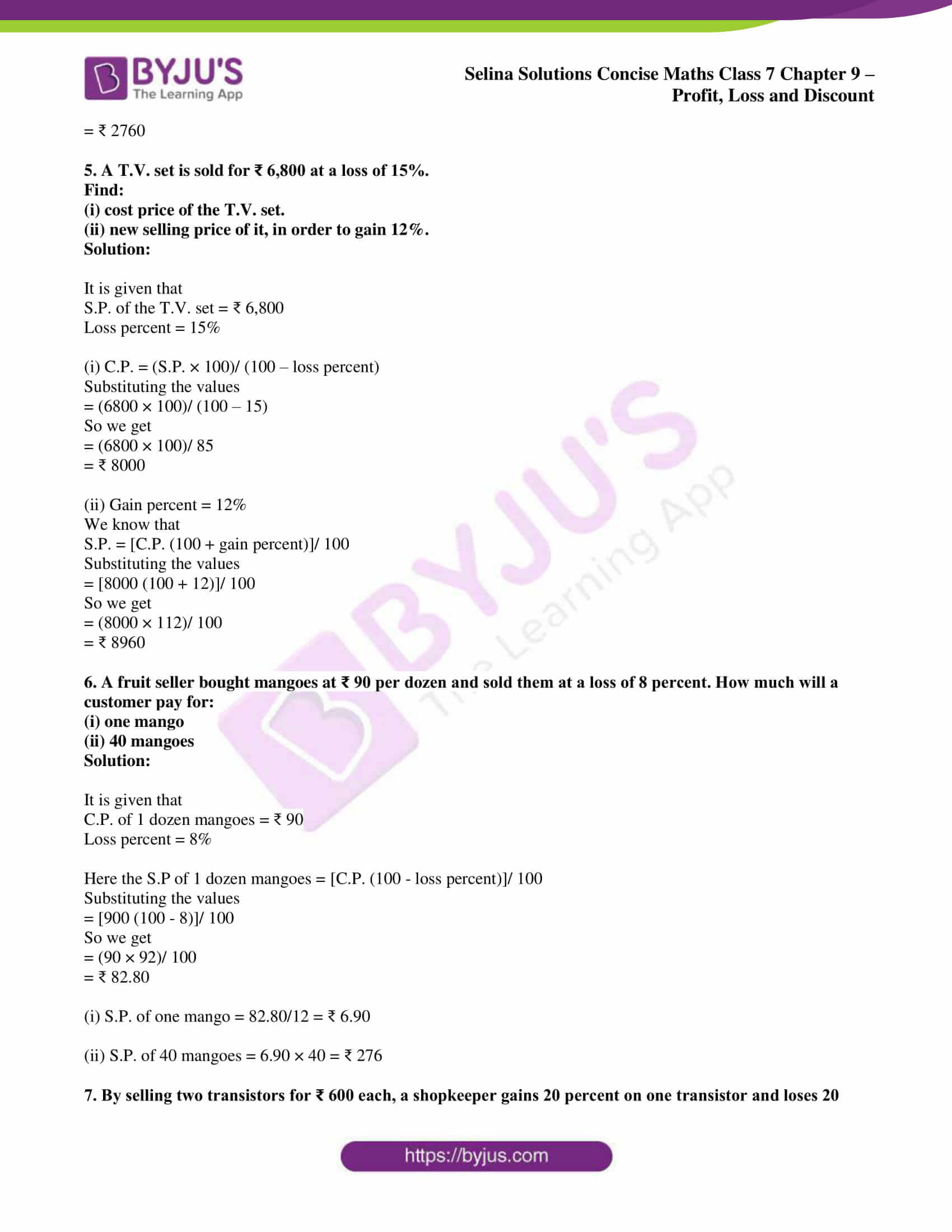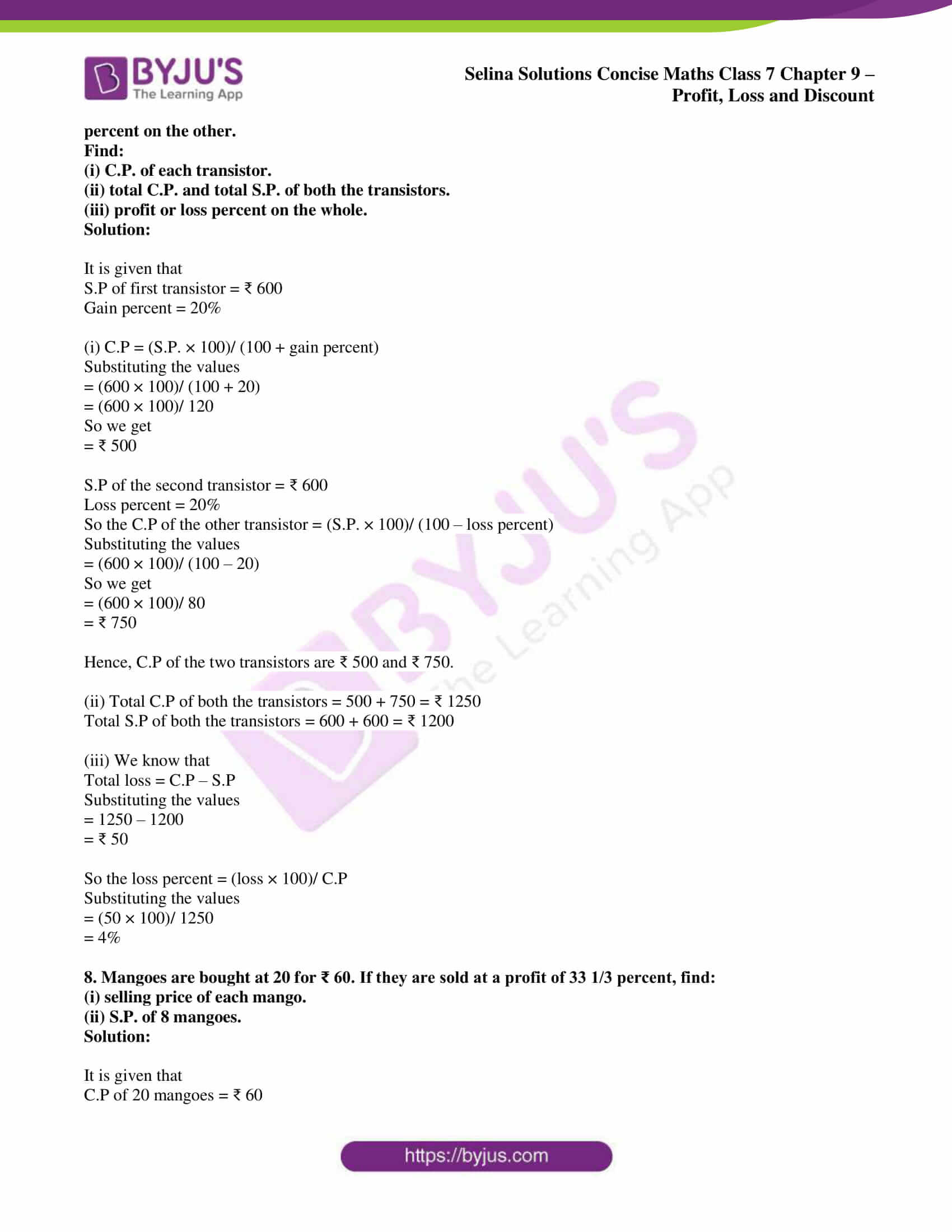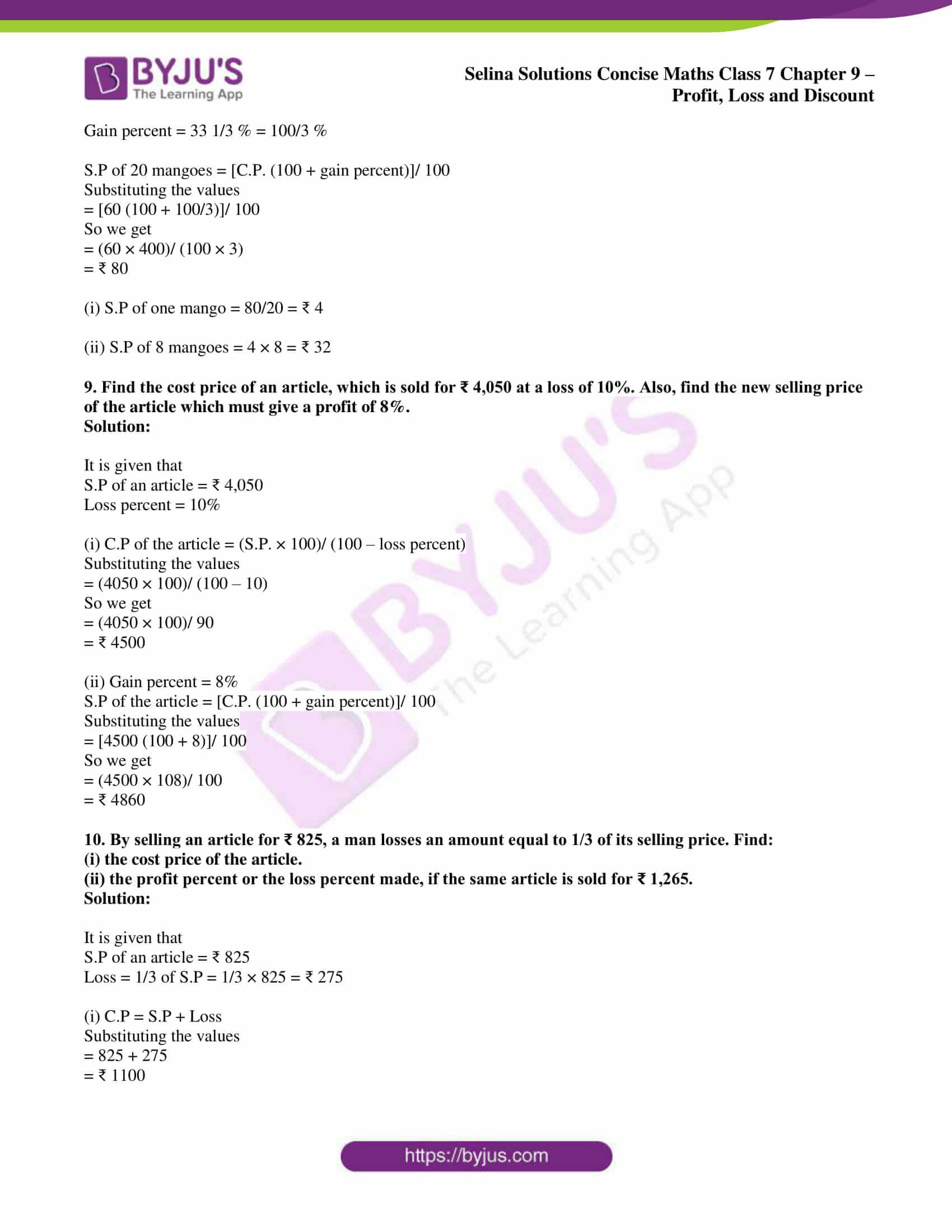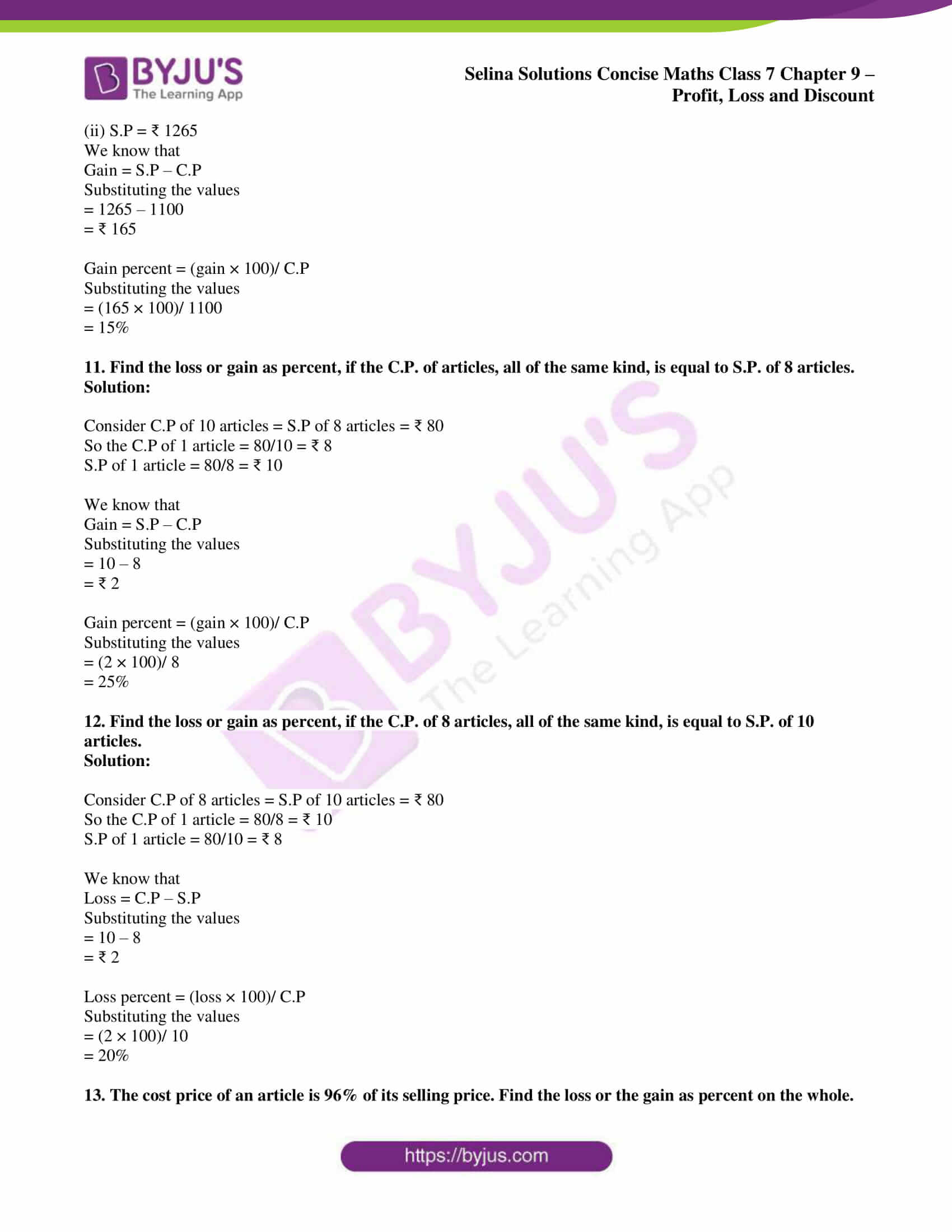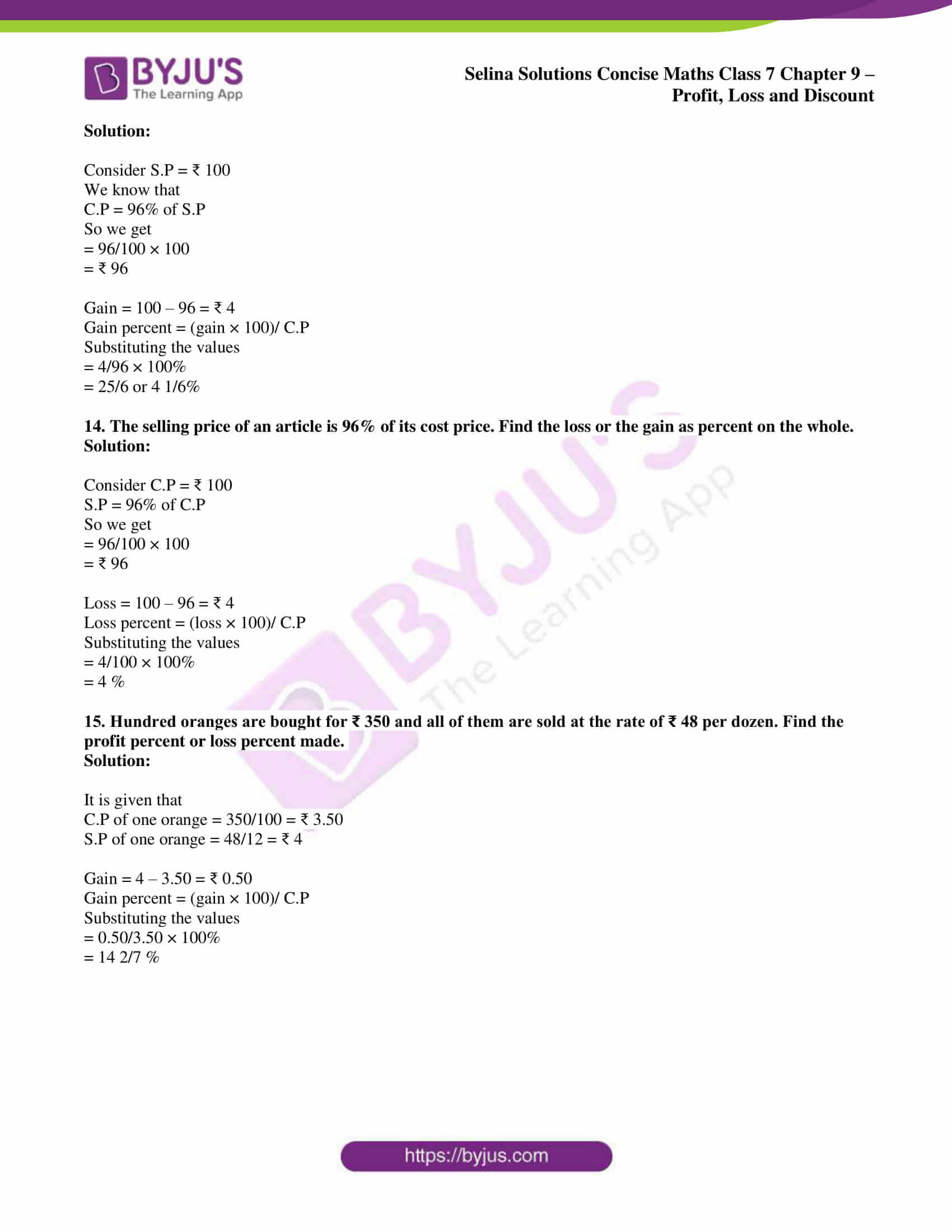### Access Selina Solutions Concise Maths Class 7 Chapter 9: Profit, Loss and Discount Exercise 9B

#### Exercise 9B page: 107

1. Find the cost price, if:

(i) S.P. = ₹ 21 and gain = 5%

(ii) S.P. = ₹ 22 and loss = 12%

(iii) S.P. = ₹ 340 and gain = ₹ 20

(iv) S.P. = ₹ 200 and loss = ₹ 50

(v) S.P. = ₹ 1 and loss = 5 p

Solution:

(i) S.P. = ₹ 21 and gain = 5%

We know that

C.P. = (S.P. × 100)/ (100 + gain percent)

Substituting the values

= (21 × 100)/ (100 + 5)

= (21 × 100)/ 105

So we get

= ₹ 20

(ii) S.P. = ₹ 22 and loss = 12%

We know that

C.P. = (S.P. × 100)/ (100 – loss percent)

Substituting the values

= (22 × 100)/ (100 – 12)

= (22 × 100)/ 88

So we get

= ₹ 25

(iii) S.P. = ₹ 340 and gain = ₹ 20

We know that

C.P. = S.P. – Gain

Substituting the values

= 340 – 20

= ₹ 320

(iv) S.P. = ₹ 200 and loss = ₹ 50

We know that

C.P. = S.P. + loss

Substituting the values

= 200 + 50

= ₹ 250

(v) S.P. = ₹ 1 and loss = 5 p

We know that

C.P. = S.P. + Loss

Substituting the values

= ₹ 1 + 5 p

= ₹ 1.05

2. By selling an article for ₹ 810, a loss of percent is suffered. Find its cost price.

Solution:

It is given that

S.P of an article = ₹ 810

Loss percent = 10 %

We know that

C.P. = (S.P. × 100)/ (100 – loss percent)

Substituting the values

= (810 × 100)/ (100 – 10)

So we get

= (810 × 100)/ 90

= ₹ 900

3. By selling a scooter for ₹ 9,200, a main gains 15%. Find the cost price of the scooter.

Solution:

It is given that

S.P. of the scooter = ₹ 9,200

Gain percent = 15%

We know that

C.P. = (S.P. × 100)/ (100 + gain percent)

Substituting the values

= (9200 × 100)/ (100 + 15)

= (9200 × 100)/ 115

So we get

= ₹ 8000

4. On selling an article for ₹ 2,640, a profit of 10 percent is made.

Find:

(i) cost price of the article.

(ii) new selling price of it, in order to gain 15%.

Solution:

It is given that

S.P. of an article = ₹ 2,640

Gain percent = 10%

(i) C.P. = (S.P. × 100)/ (100 + gain percent)

Substituting the values

= (2640 × 100)/ (100 + 10)

= (2640 × 100)/ 110

So we get

= ₹ 2400

(ii) Gain percent = 15%

S.P. = [C.P. (100 + gain percent)]/ 100

Substituting the values

= [2400 (100 + 15)]/ 100

So we get

= (2400 × 115)/ 100

= ₹ 2760

5. A T.V. set is sold for ₹ 6,800 at a loss of 15%.

Find:

(i) cost price of the T.V. set.

(ii) new selling price of it, in order to gain 12%.

Solution:

It is given that

S.P. of the T.V. set = ₹ 6,800

Loss percent = 15%

(i) C.P. = (S.P. × 100)/ (100 – loss percent)

Substituting the values

= (6800 × 100)/ (100 – 15)

So we get

= (6800 × 100)/ 85

= ₹ 8000

(ii) Gain percent = 12%

We know that

S.P. = [C.P. (100 + gain percent)]/ 100

Substituting the values

= [8000 (100 + 12)]/ 100

So we get

= (8000 × 112)/ 100

= ₹ 8960

6. A fruit seller bought mangoes at ₹ 90 per dozen and sold them at a loss of 8 percent. How much will a customer pay for:

(i) one mango

(ii) 40 mangoes

Solution:

It is given that

C.P. of 1 dozen mangoes = ₹ 90

Loss percent = 8%

Here the S.P of 1 dozen mangoes = [C.P. (100 – loss percent)]/ 100

Substituting the values

= [900 (100 – 8)]/ 100

So we get

= (90 × 92)/ 100

= ₹ 82.80

(i) S.P. of one mango = 82.80/12 = ₹ 6.90

(ii) S.P. of 40 mangoes = 6.90 × 40 = ₹ 276

7. By selling two transistors for ₹ 600 each, a shopkeeper gains 20 percent on one transistor and loses 20 percent on the other.

Find:

(i) C.P. of each transistor.

(ii) total C.P. and total S.P. of both the transistors.

(iii) profit or loss percent on the whole.

Solution:

It is given that

S.P of first transistor = ₹ 600

Gain percent = 20%

(i) C.P = (S.P. × 100)/ (100 + gain percent)

Substituting the values

= (600 × 100)/ (100 + 20)

= (600 × 100)/ 120

So we get

= ₹ 500

S.P of the second transistor = ₹ 600

Loss percent = 20%

So the C.P of the other transistor = (S.P. × 100)/ (100 – loss percent)

Substituting the values

= (600 × 100)/ (100 – 20)

So we get

= (600 × 100)/ 80

= ₹ 750

Hence, C.P of the two transistors are ₹ 500 and ₹ 750.

(ii) Total C.P of both the transistors = 500 + 750 = ₹ 1250

Total S.P of both the transistors = 600 + 600 = ₹ 1200

(iii) We know that

Total loss = C.P – S.P

Substituting the values

= 1250 – 1200

= ₹ 50

So the loss percent = (loss × 100)/ C.P

Substituting the values

= (50 × 100)/ 1250

= 4%

8. Mangoes are bought at 20 for ₹ 60. If they are sold at a profit of 33 1/3 percent, find:

(i) selling price of each mango.

(ii) S.P. of 8 mangoes.

Solution:

It is given that

C.P of 20 mangoes = ₹ 60

Gain percent = 33 1/3 % = 100/3 %

S.P of 20 mangoes = [C.P. (100 + gain percent)]/ 100

Substituting the values

= [60 (100 + 100/3)]/ 100

So we get

= (60 × 400)/ (100 × 3)

= ₹ 80

(i) S.P of one mango = 80/20 = ₹ 4

(ii) S.P of 8 mangoes = 4 × 8 = ₹ 32

9. Find the cost price of an article, which is sold for ₹ 4,050 at a loss of 10%. Also, find the new selling price of the article which must give a profit of 8%.

Solution:

It is given that

S.P of an article = ₹ 4,050

Loss percent = 10%

(i) C.P of the article = (S.P. × 100)/ (100 – loss percent)

Substituting the values

= (4050 × 100)/ (100 – 10)

So we get

= (4050 × 100)/ 90

= ₹ 4500

(ii) Gain percent = 8%

S.P of the article = [C.P. (100 + gain percent)]/ 100

Substituting the values

= [4500 (100 + 8)]/ 100

So we get

= (4500 × 108)/ 100

= ₹ 4860

10. By selling an article for ₹ 825, a man losses an amount equal to 1/3 of its selling price. Find:

(i) the cost price of the article.

(ii) the profit percent or the loss percent made, if the same article is sold for ₹ 1,265.

Solution:

It is given that

S.P of an article = ₹ 825

Loss = 1/3 of S.P = 1/3 × 825 = ₹ 275

(i) C.P = S.P + Loss

Substituting the values

= 825 + 275

= ₹ 1100

(ii) S.P = ₹ 1265

We know that

Gain = S.P – C.P

Substituting the values

= 1265 – 1100

= ₹ 165

Gain percent = (gain × 100)/ C.P

Substituting the values

= (165 × 100)/ 1100

= 15%

11. Find the loss or gain as percent, if the C.P. of articles, all of the same kind, is equal to S.P. of 8 articles.

Solution:

Consider C.P of 10 articles = S.P of 8 articles = ₹ 80

So the C.P of 1 article = 80/10 = ₹ 8

S.P of 1 article = 80/8 = ₹ 10

We know that

Gain = S.P – C.P

Substituting the values

= 10 – 8

= ₹ 2

Gain percent = (gain × 100)/ C.P

Substituting the values

= (2 × 100)/ 8

= 25%

12. Find the loss or gain as percent, if the C.P. of 8 articles, all of the same kind, is equal to S.P. of 10 articles.

Solution:

Consider C.P of 8 articles = S.P of 10 articles = ₹ 80

So the C.P of 1 article = 80/8 = ₹ 10

S.P of 1 article = 80/10 = ₹ 8

We know that

Loss = C.P – S.P

Substituting the values

= 10 – 8

= ₹ 2

Loss percent = (loss × 100)/ C.P

Substituting the values

= (2 × 100)/ 10

= 20%

13. The cost price of an article is 96% of its selling price. Find the loss or the gain as percent on the whole.

Solution:

Consider S.P = ₹ 100

We know that

C.P = 96% of S.P

So we get

= 96/100 × 100

= ₹ 96

Gain = 100 – 96 = ₹ 4

Gain percent = (gain × 100)/ C.P

Substituting the values

= 4/96 × 100%

= 25/6 or 4 1/6%

14. The selling price of an article is 96% of its cost price. Find the loss or the gain as percent on the whole.

Solution:

Consider C.P = ₹ 100

S.P = 96% of C.P

So we get

= 96/100 × 100

= ₹ 96

Loss = 100 – 96 = ₹ 4

Loss percent = (loss × 100)/ C.P

Substituting the values

= 4/100 × 100%

= 4 %

15. Hundred oranges are bought for ₹ 350 and all of them are sold at the rate of ₹ 48 per dozen. Find the profit percent or loss percent made.

Solution:

It is given that

C.P of one orange = 350/100 = ₹ 3.50

S.P of one orange = 48/12 = ₹ 4

Gain = 4 – 3.50 = ₹ 0.50

Gain percent = (gain × 100)/ C.P

Substituting the values

= 0.50/3.50 × 100%

= 14 2/7 %